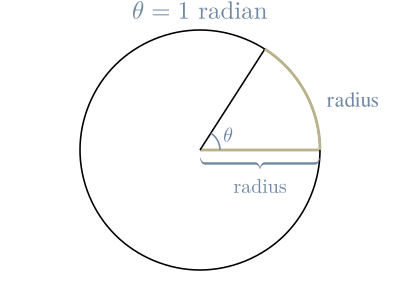A radian is a measurement of an angle.

It measures the number of radiuses that "subtends" the angle. In other words, the count of radiuses on the side opposite the angle.

It is an alternative to measuring angles in degrees.

A full rotation, 360°, is the circumference of a circle. Therefore, $2 \ \pi \ \textrm{radians} = 360°$.

To calculate 180°, we can divide both sides by 2: $\frac{2 \ \pi \ \textrm{radians}}{2} = \frac{360°}{2}$ which gives us $\pi \ \textrm{radians} = 180°$

We can then divide both sides by $\pi$ to find one radian: $\text{radian} = \frac{180}{\pi} = ~57.2958$Most programming languages provide simple functions for converting between radians and degrees.